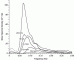I have forgotten

•http://facebook.com/
•https://www.google.com/accounts/o8/id
•https://me.yahoo.com

# Spectra

#### JONSWAP

The JONSWAP spectra in the wave-frequency domain
 double JONSWAP_Gnnw (double w, double wp, double alpha=0.0081, double gamma=3.3, double beta=1.25) double JONSWAP_wp (double wind, double length) double JONSWAP_alpha (double wind, double length) double JONSWAP_Gnnk (double k, double wp, double depth=0, double alpha=0.0081,double gamma=3.3, double beta=1.25)

#### Pierson Moskowitz

Defines the Pierson Moskowitz spectra in the wave-frequency domain
 double PM_Gnnw_U (double w, double U, double alpha=0.0081, double beta=1.25) double PM_Gnnw_wp (double w, double wp, double alpha=0.0081, double beta=1.25) double PM_Gnnw (double w, double wp, double alpha=0.0081, double beta=1.25) double PM_Gnnw_Tp (double w, double Hs, double Tp) double PM_Gnnw_Tz (double w, double Hs, double Tz) double PM_wind_to_wp (double wind) double PM_wind_to_Tp (double wind) double PM_Tp_to_alpha (double Hs, double Tp) double PM_Tz_to_alpha (double Hs, double Tz) double PM_Tz_to_Tp (double Tz) double PM_Gnnk_wp (double k, double wp, double depth=0, double alpha=0.0081, double beta=1.25) double PM_Gnnk (double k, double wp, double depth=0, double alpha=0.0081, double beta=1.25)